# MINT Microbial Case Study

This case study will focus on applying the P-integrative framework (MINT) to a set of microbial data, derived from two different studies. The ultimate goal is to generate a sPLS-DA model which can determine what inhibiting agent a given microbiome inhabitant has been affected by based on signals present in OTU (Operational Taxonomic Unit) readings.

# Rscript

The R script used for all the analysis in this case study is available here.

# To begin

Load the latest version of mixOmics.

library(mixOmics) # import the mixOmics library


## The data

The data being used in the case study derives from anaerobic respiration inhibition research. There are a total of four studies included in this data [Poirier S. et al., 2018(a), Poirier S. et al., 2018(b)], measured across the same set of OTUs. They were compiled and analysed in conjunction [Poirier S. et al., 2020]. Certain microbiome inhabitants can have their anaerobic respiration inhibited by the presence of certain substrates. These studies focused on the impact of ammonia (NH4) on this process.

Download the data here. The data_prediction_data.RData file contains the following dataframes:

• metadata_studies_1_2 (continuous and categotical matrix): 55 rows and 40 columns. Contains much of the metadata for each of the samples for studies 1 and 2. For this case study, only variables used from this dataframe is the experiment feature (denotes which study each sample is drawn from) and inhib_inoc feature (denotes the type of inhibition for that sample).
• metadata_studies_3_4 (continuous and categotical matrix): 46 rows and 40 columns. Contains much of the metadata for each of the samples for studies 3 and 4. For this case study, only variables used from this dataframe is the experiment feature (denotes which study each sample is drawn from) and inhib_inoc feature (denotes the type of inhibition for that sample).
• abundance_studies_1_2 (continuous matrix): 51 rows and 55 columns. Contains the raw OTU (rows) values for each of the samples (columns) for studies 1 and 2.
• abundance_studies_3_4 (continuous matrix): 51 rows and 46 columns. Contains the raw OTU (rows) values for each of the samples (columns) for studies 3 and 4.
load("Microbial Data/data_prediction_data.RData") # load the data

# extract the Y vectors
treatment.1.2 <- metadata_studies_1_2$inhib_inoc treatment.3.4 <- metadata_studies_3_4$inhib_inoc
treatment <- c(treatment.1.2, treatment.3.4)

# extract the study each sample is from
study.1.2 <- metadata_studies_1_2$experiment study.3.4 <- metadata_studies_3_4$experiment
study <- c(study.1.2,study.3.4)

# combine abundance datasets into a single dataframe
abundance <- cbind(abundance_studies_1_2, abundance_studies_3_4)


## Pre-processing the data

While this case study is not using the mixMC framework, the concepts from MixMC Pre-processing are still valid as the data is microbial in nature. Hence, the same steps outlined in that page will be followed here.

First an offset was applied to prevent the presence of any zeroes. Then, features with significantly low counts were to be removed to avoid any spurious conclusions – however none fell below the threshold and therefore none were removed. Finally, a CLR (centered log ratio) transformation was applied.

## Data exploration

Now that the data is processed, it is ready for analysis. As with all other case studies on the site, prior to diving into analysis with the method believed to be the most appropriate, exploring the data with simpler methods can save much time down the track. Hence, the data will go through a PCA and a standard sPLS-DA before the MINT framework is applied.

### PCA

Looking at the projection of the samples in the PC space (Figure 1), the samples from different study are more or less separated. This is a clear example of a batch effect – a bias in the data where the distribution differences across studies outweighs the distribution differences between classes. This is exactly what needs to be controlled by the MINT methodology. Figure 1 suggests the classes can be separated, but the significant overlap of the confidence ellipses indicates that a much better model can be developed.

# undergo PCA
ab.pca <- pca(abundance.processed, scale = TRUE, center = TRUE, ncomp = 5)

# plot projection of samples in PC space
plotIndiv(ab.pca, group=treatment,
ind.names = F,legend=T,
pch = as.numeric(factor(study))+14,
pch.levels=(study), ellipse = TRUE,
title="PCA",legend.title = "Inhibitor",
legend.title.pch = "Experiment", size.legend = rel(2.4),
size.legend.title = rel(2.5))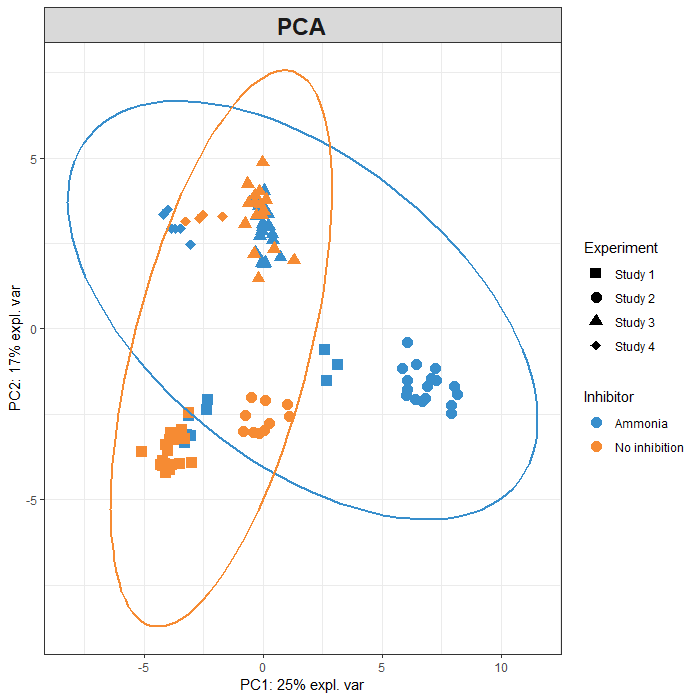FIGURE 1: Projection of the samples from studies 1 and 2 onto the first two principal components.

## MINT sPLS-DA Model

Now that the data have been explored, and the experimental effect identified, the next step involves the use of the MINT framework. Prior to this however, the data shall be once again split. The first two studies will be used as training data while the third and fourth studies are to be used as a validation set.

# determine indices of training and testing sets
train.idx <- which(study %in% c("Study 1", "Study 2"))
test.idx <- which(study %in% c("Study 3", "Study 4"))

# separate the predictors
X.train <- abundance.processed[train.idx, ]
X.test <- abundance.processed[test.idx, ]

# separate the class labels
Y.train <- treatment[train.idx]
Y.test <- treatment[test.idx]

# separate the study associations
study.train <- as.factor(as.character(study[train.idx]))
study.test <- as.factor(as.character(study[test.idx]))


### Tuning the model

Using just the training data, the model is first tuned. Observing the error rates for each keepX on each component, the minimum is achieved by keepX = 17 on the first component. Addition of the second to fifth components actually make no improvements over a model with one component. However, as visualisation is desired, a minimum component count of 2 will be used. Note that the lines depicting components 3 and 4 are not visible as they are the exact same as the fifth component (which is rendered on top of these two).

# tune the ncomp and keepX parameters for the MINT sPLS-DA model
ab.mint.splsda.tuning <- tune(method = "mint.splsda",
X = X.train,
Y = Y.train,
study = study.train,
ncomp = 5,
test.keepX = seq(5,50, 3),
measure = 'BER', # balanced error rate
dist = "centroids.dist")

plot(ab.mint.splsda.tuning, sd = FALSE) # sd = F due to LOO CV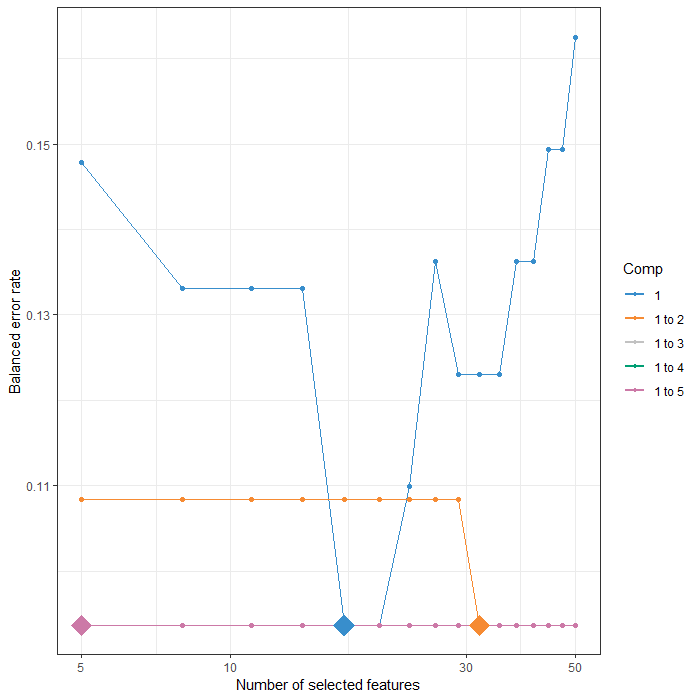FIGURE 2: Tuning plot of the MINT sPLS-DA models with up to 5 components. Diamonds represent the optimal number of features on a given component. Balanced error rate found on the vertical axis and is the metric to be minimised.

optimal.ncomp <- 2

# extract the optimal keepX parameter
optimal.keepX <- ab.mint.splsda.tuning\$choice.keepX[1:optimal.ncomp]

optimal.keepX

## comp1 comp2
##    17    32


### Training the model on studies 1 and 2

Using the tuned parameter values, a model is generated and trained on the first two studies.

# form tuned, trained MINT sPLS-DA model
ab.mint.splsda <- mint.splsda(X = X.train, Y = Y.train,
ncomp = optimal.ncomp, keepX = optimal.keepX,
study = study.train)


### Visualising the model

#### Sample Plots

As always, the plot to start with is the sample projection plot. Figure 3 depicts the projection of the samples onto the 'global' variates, that which represent all studies. The placebo group has the studies separated along the first component while the studies are separated along the second component for the ammonia samples. Hence, the batch effect is still slightly present, but its impacts within each class have been severely reduced. The class distinction is significantly better than that of Figure 1 as they are linearly separable with the confidence ellipses sharing no overlap.

plotIndiv(ab.mint.splsda, ind.names = F,legend=T,
pch = as.numeric(factor(study.train))+14,
pch.levels=study.train,
ellipse = T,
subtitle="sPLS-DA Sample Projection",legend.title = "Inhibitor",
legend.title.pch = "Experiment",
size.legend = rel(0.8))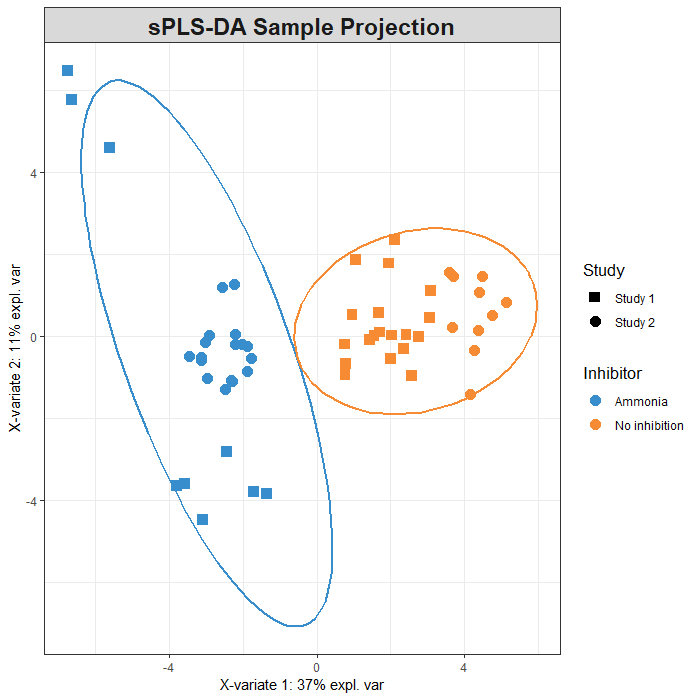FIGURE 3: Projection of the samples from both studies onto the global variates yielded by MINT sPLS-DA.

#### Variable Plots

Understanding how the model has constructed the variates is an important step in learning how these classes are distinct. When considering global component 1 (Figure 4(a)), the association of each variable with a specific class is clear. Positively contributing features are exclusively associated with the no inhibition class while all negatively contributing features defined the ammonia group. This suggests that the first component (in the global space) is key in discriminating between these two classes. The functionality of the second component is not as clear, but Figure 4(b) does show the features which are the most important to this component.

plotLoadings(ab.mint.splsda, method = "median", comp = 1,
legend = T, study = "global", contrib = "max",
title = "(a) All Studies, Comp 1")

legend = T, study = "global", contrib = "max",
title = "(b) All Studies, Comp 2")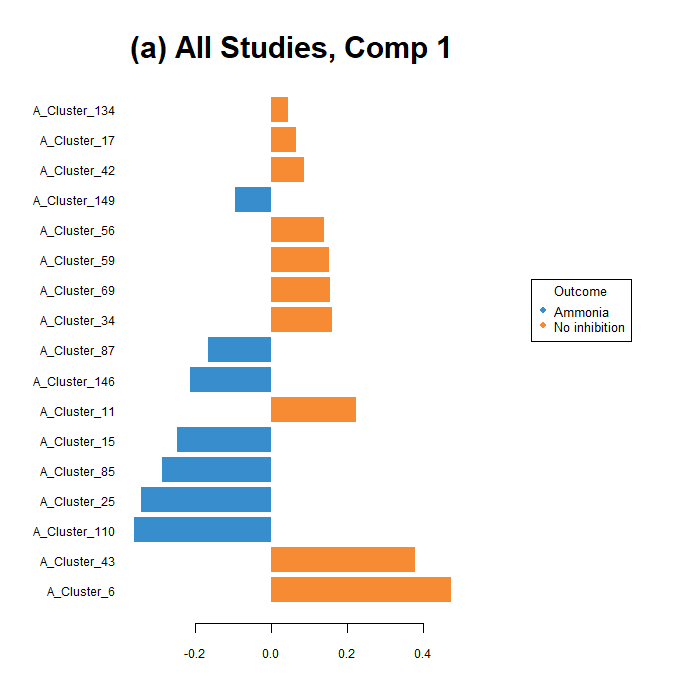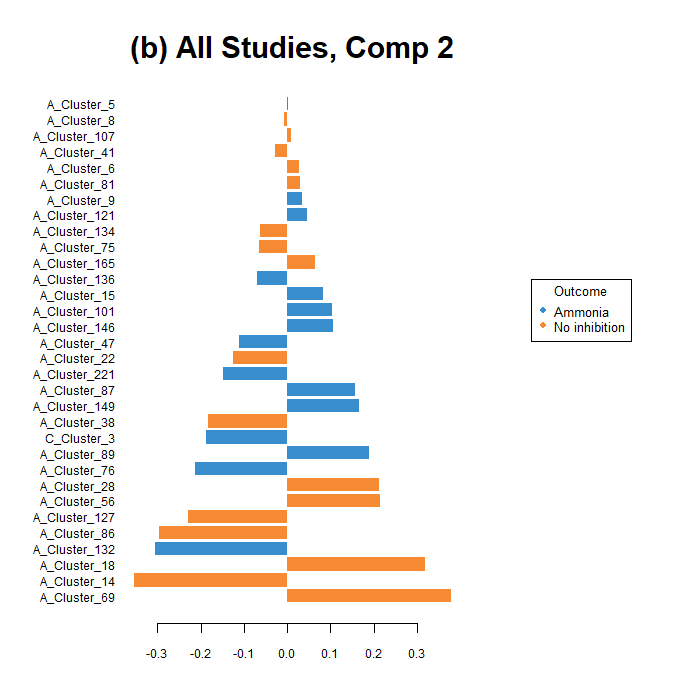FIGURE 4: Feature loading values on the global, first (a) and second (b) component. Bars coloured based on the treatment class with the highest median on that variable.

The correlation circle plot (Figure 5) shows that a majority of the selected features have strong associations (both positive and negative) with the first component. There is a smaller set of those which define the second component. Those on the negative side of the first component are more tightly clustered, suggesting features that define the ammonia class are more homogeneous and the placebo group has signals that are more disparate.

plotVar(ab.mint.splsda, var.names = FALSE,
pch = 16, cutoff = 0.5)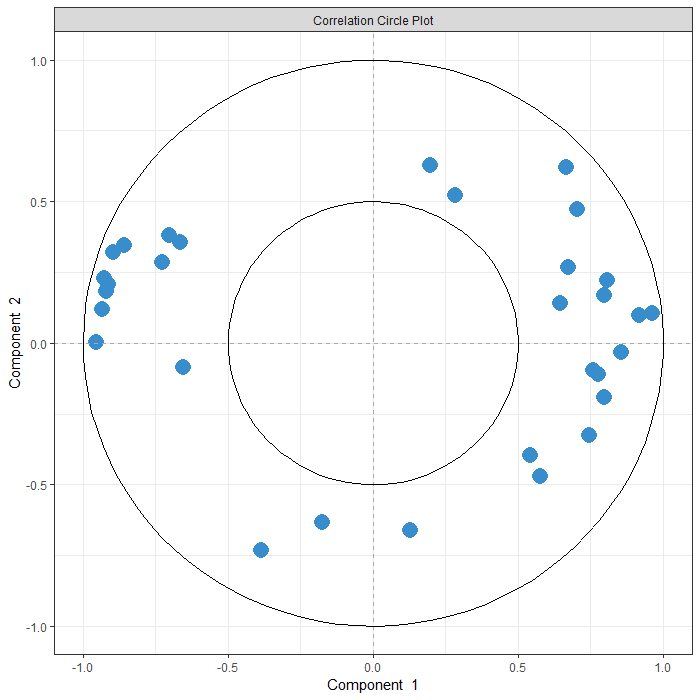FIGURE 5: Correlation circle plot representing the OTUs selected by MINT sPLS-DA performed on the microbial data to examine the association of the selected OTUs on the first two components. A minimum correlation value of 0.5 is required to be depicted on these axes.

The below CIM visualises the filtered and transformed abundance data across all the selected OTUs. There are a few 'blocks' of homogeneous abundance counts. For instance, the placebo group has (on average) higher abundances for those OTUs in the right-most cluster while the ammonia class has higher abundances for those in the left cluster. The clustering of the rows shows the usefulness of the MINT framework. All the samples belonging to the same class were clustered together, irrespective of what study they were drawn from. This 'perfect' class clustering is not achieved by the standard sPLS-DA model.

cim(ab.mint.splsda,
row.sideColors = cbind(color.mixo(as.numeric(Y.train)),
color.mixo(as.numeric(study.train)+4)),

legend = list(legend = cbind(c(levels(Y.train)), c(levels(study.train))),
col = cbind(c(color.mixo(1:2)), c(color.mixo(5:6))),
title = "Treatment and Study", cex = 0.8)
)FIGURE 6: Clustered Image Map of the OTUs selected by MINT sPLS-DA on the microbial data using all three components. A hierarchical clustering based on the counts of the selected OTUs was used, with samples in rows coloured according to the inoculation method.

Lastly, the relevance network plot can be used to further understand the relationship between variables and classes. Note that cutoff = 0.7, such that any correlations below this value are not displayed at all. Being unable to control the position of the nodes in this plot makes interpretation more difficult. However, a key takeaway from this network is that all features with strong correlations (ie. above 0.7) have a positive association with one of the two classes and a negative association with the other.

network(ab.mint.splsda, cutoff = 0.7, comp = 1,
color.node = c(color.mixo(1), color.mixo(2)),
shape.node = c("circle", "rectangle"),
lty.edge = c("dotted", "solid"),
cex.node.name = 0.7,
alpha.node = 0.5,
row.names = names)FIGURE 7: Relevance network of the selected features on the first component by MINT sPLS-DA performed on the microbial OTU data. Dotted, red lines are positive while solid, green lines are negative. Minimum correlation value of 0.7 required to be shown. Number circles denote specific OTUs (number specifies which OTU) while rectangles denote classes.

### Testing the model on studies 3 and 4

After the model has been explored, it's performance as a classifier is to be assessed. Here, the perf() function is used to determine which distance metric will be most appropriate to use. Addition of the second component either has no impact on the model performance, or in the case of the mahalanobis distance, worsened performance. Based on Figure 8, classification error rate is minimised using max.dist.

ab.mint.splsda.perf <- perf(ab.mint.splsda, folds = 5, nrepeat = 10)
plot(ab.mint.splsda.perf)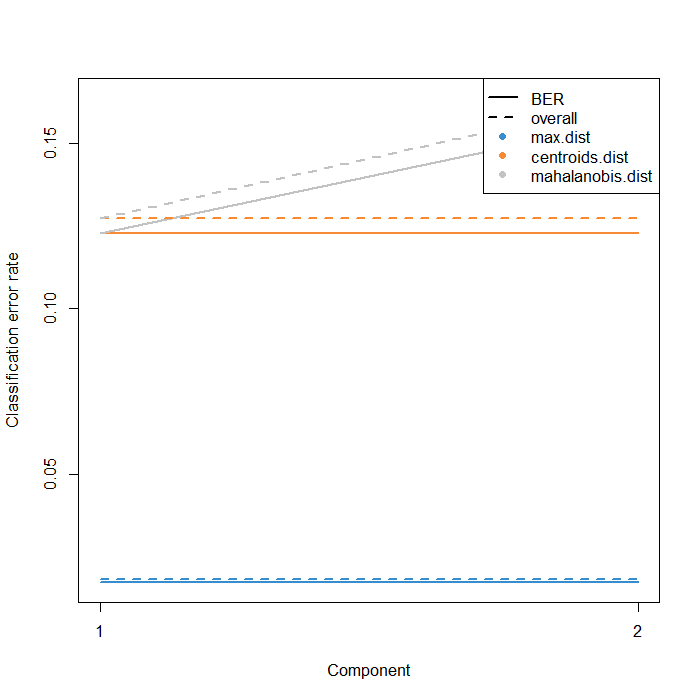FIGURE 8: Classification error rate of the MINT sPLS-DA models, using up to two components for all three distance metrics.

Using the model generated above and the predict() function, predictions of the class labels for all samples from studies 3 and 4 can be made.

# make predictions of stem cell type of study 3 samples
predict.splsda <- predict(ab.mint.splsda, newdata = X.test,
dist = "max.dist",
study.test = study.test)


The below custom function YieldErrorRates() merely calculates the confusion matrix based on the predictions of the model and then returns the error rate and balanced error rate for a model using a given number of components.

As can be seen below, the error rate and balanced error both decrease with the addition of the second component, though the model was already quite good at classifying these novel samples with just one component. This is unsurprising as looking at Figure 3, the first component separated the two classes entirely.

YieldErrorRates(1)

## Metrics for model with 1 component(s):
## Error rate:  0.173913
## Balanced error rate:  0.1711538

YieldErrorRates(2)

## Metrics for model with 2 component(s):
## Error rate:  0.08695652
## Balanced error rate:  0.09423077


Below, Figure 9 goes to show that sometimes the AUROC plot can show off some optimistic results. For models using one or both components the AUC is 1 despite the fact that the error rate was non-zero. This is also a good example of why evaluating a model using a variety of metrics (ie. error rate, specificity, recall, etc) is important as they don't always agree. In this case, the overall error rate is the most useful though this may not always be the case.

auroc(ab.mint.splsda, roc.comp = 2, print = FALSE)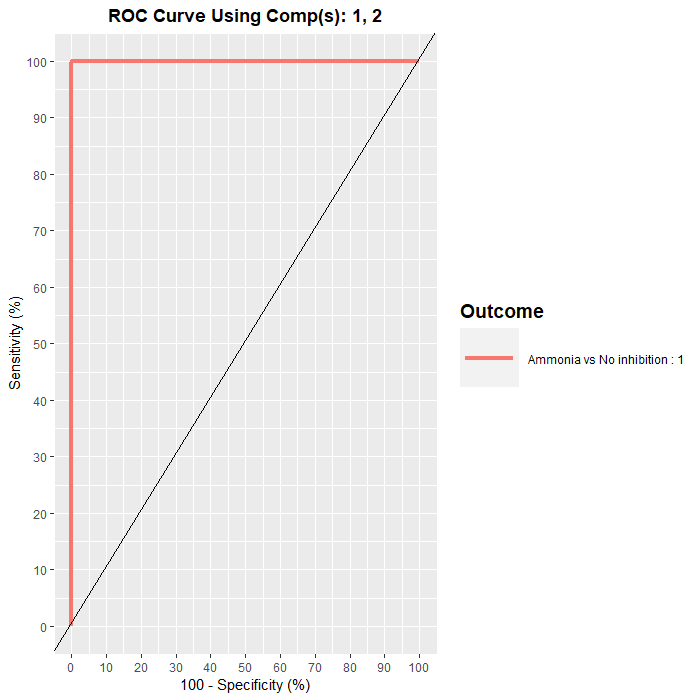FIGURE 9: ROC curve and AUC from the MINT sPLS-DA performed on the microbial OTU data using both components. Numerical output on right is the AUC values for the model.